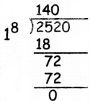Students can download 5th Maths Term 3 Chapter 5 Money Ex 5.2 Questions and Answers, Notes, Samacheer Kalvi 5th Maths Guide Pdf helps you to revise the complete Tamilnadu State Board New Syllabus, helps students complete homework assignments and to score high marks in board exams.

## Tamilnadu Samacheer Kalvi 5th Maths Solutions Term 3 Chapter 5 Money Ex 5.2

Question 1.
Fill in the blanks

(i) ₹ 75 × 5 = ________
375

(ii) ₹ 200.25 ÷ 25 = ________
₹ 8.01(iii) ₹ 3500 ÷ 500 = ________
₹ 7

(iv) ₹ 15.50 × 100 = ________
₹ 1550

Question 2.
(i) ₹ 98725 × 5(ii) ₹ 679.68 × 7(iii) 362.37 × 12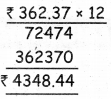(iv) 324.52 ÷ 28(v) 7980 ÷ 8(vi) 397.10 ÷ 11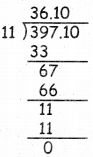Question 3.
The cost of 1 kg of tomato is ₹ 15. Find the cost of 5 kg of tomatoes?
Cost of 1 kg = ₹ 15
Cost of 5 kg = ₹ 15 × 5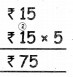Question 4.
The cost of one egg is ₹ 4.50. Find the cost of 20 eggs.
Cost of 1 egg = ₹ 4.50
Cost of 20 egg = ₹ 4.50 × 20
= ₹ 90.00Question 5.
The school management has decided to give a pen for all children participating in the Children’s day celebration. The cost of a pen is
₹ 18. How much money do they need to buy pens for 256 children?
Cost of a pen = ₹ 18
Number of children = 256
Money needed = 256 × 18Question 6.
A fruit seller buys 8 boxes ef grapes for ₹ 2,000. What is the cost of one box?
Cost of 1 boxes = $$\frac { 2000 }{ 8 }$$ = 250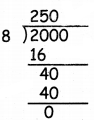Cost of 1 kg = $$\frac { 2520 }{ 18 }$$ = ₹ 140